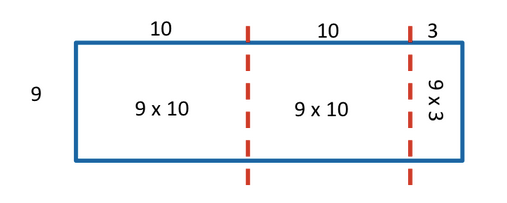# Grade 4 Math: Distributive Property and Open Arrays

Dear Families,

I know you have been seeing homework involving the distributive property and open arrays.  It may seem a little foreign since most of us never learned about arrays when we were in school.  Arrays are a great visual model for representing multiplication and representing the distributive property.

If you want to solve a problem like 23 x 9 without using an algorithm, you can think about breaking apart one or more of the numbers and multiplying the parts.  This is essentially the distributive property.  What’s great about solving in this way is that you can break apart the numbers in whatever way you choose.  Often students decompose the numbers into tens and ones, but sometimes they just break them up into facts they know.

Here is an example of one way to use the distributive property to solve 9 x 23 displayed on the open array:In this case, the students decomposed the 23 into 10+10+3 and turned 9 x 23 into 9 x (10 + 10 + 3).  Then they can multiply the parts and add up the products: (9×10) + (9×10) + (9×3) = 90 + 90 + 27= 207.   If students are more comfortable with multiplying by multiples of 10, they may also decompose the 23 into 20+3.  There are a multiple options (pun intended).

Next week, we will be applying this concept to double digit by double digit multiplication.  Have a great weekend!